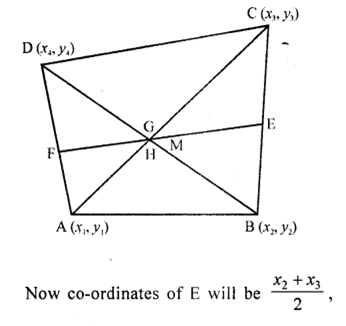• +91 9971497814
• info@interviewmaterial.com

# RD Chapter 14- Co-ordinate Geometry Ex-14.4 Interview Questions Answers

### Related Subjects

Question 1 :
Find the centroid of the triangle whose vertices are :
(i) (1, 4), (-1, -1), (3, -2)
(ii) (-2, 3), (2, -1), (4, 0)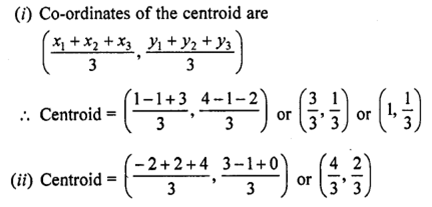Question 2 : Two vertices of a triangle are (1, 2), (3, 5) and its centroid is at the origin. Find the Co-ordinates of the third vertex.

Centroid of a triangle is O(0, 0) ….(i)
Co-ordinates of two vertices of a ∆ABC are A (1, 2) and B (3, 5)
Let the third vertex be (x, y)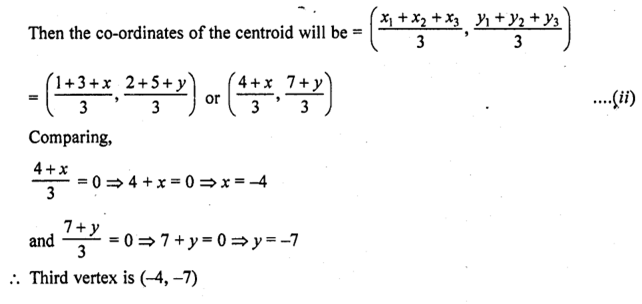Question 3 : Find the third vertex of a triangle, if two of its vertices are at (-3, 1) and (0, -2) and the centroid is at the origin.

Let two vertices of a ∆ABC be A (-3, 1) and B (0, -2) and third vertex C be (x, y)
Centroid of the ∆ABC is O (0, 0)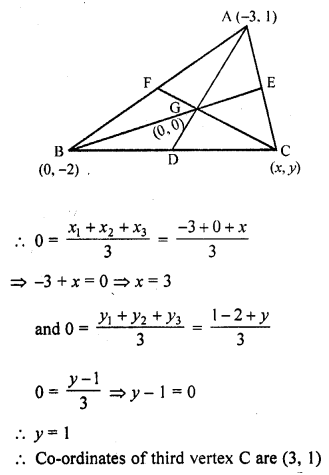Question 4 : A (3, 2) and B (-2, 1) are two vertices of a triangle ABC whose centroid G has the coordinates (5/3 , −1/3) . Find the coordinates of the third vertex C of the triangle. [CBSE 2004]

A (3, 2) and B (-2, 1) are the two vertices of ∆ABC whose centroid is G (5/3 , −1/3)
Let third vertex C be (x, y)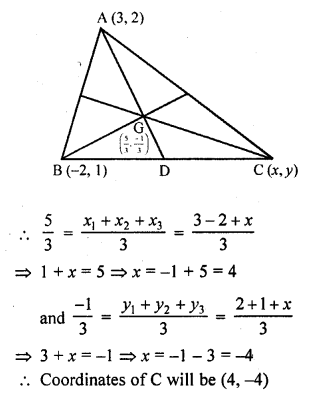Question 5 : If (-2, 3), (4, -3) and (4, 5) are the mid-points of the sides of a triangle, find the co-ordinates of its centroid.

Answer 5 : In ∆ABC, D, E and F are the mid-points of the sidesBC, CA and AB respectively.
The co-ordinates of D are (-2, 3), of E are (4,-3) and of F are (4, 5)
Let the co-ordinates of A, B and C be (x1, y1),(x2, y2), (x3, y3)respectively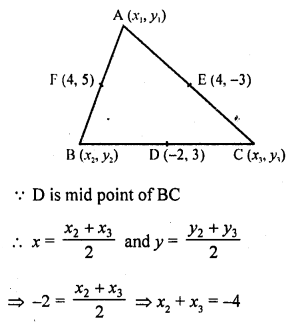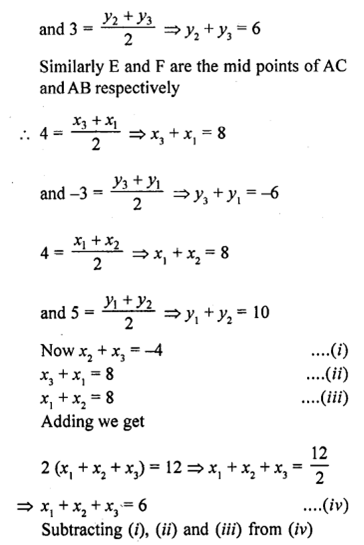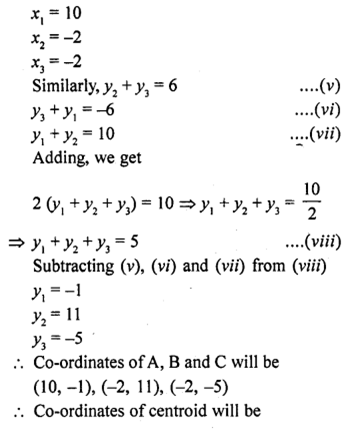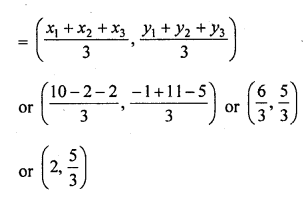Question 6 : Prove analytically that the line segment joining the middle points of two sides of a triangle is equal to half of the third side.

In ∆ABC,
D and E are the mid points of the sides AB and AC respectively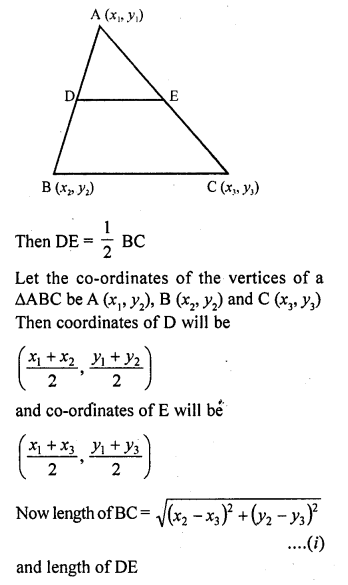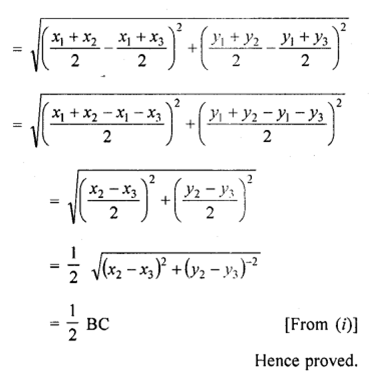Question 7 : Prove that the lines joining the middle points of the opposite sides of a quadrilateral and the join of the middle points of its diagonals meet in a point and bisect one another.